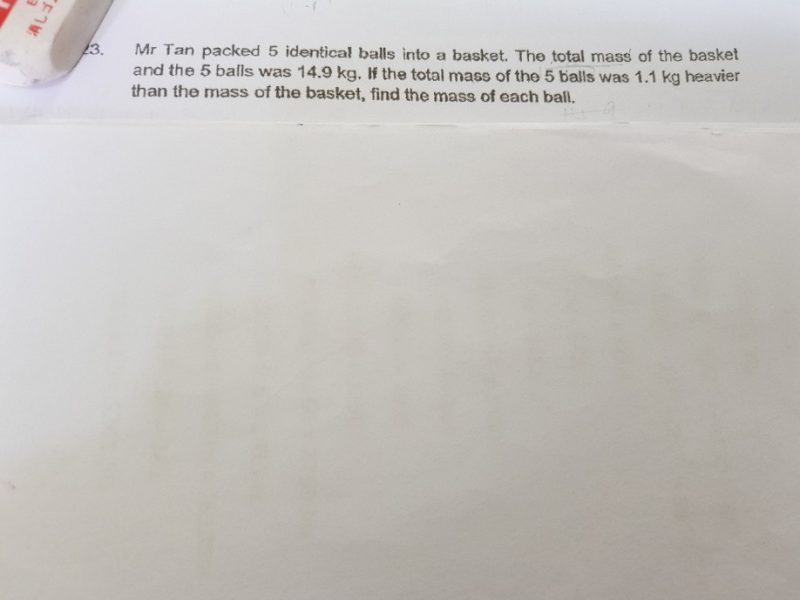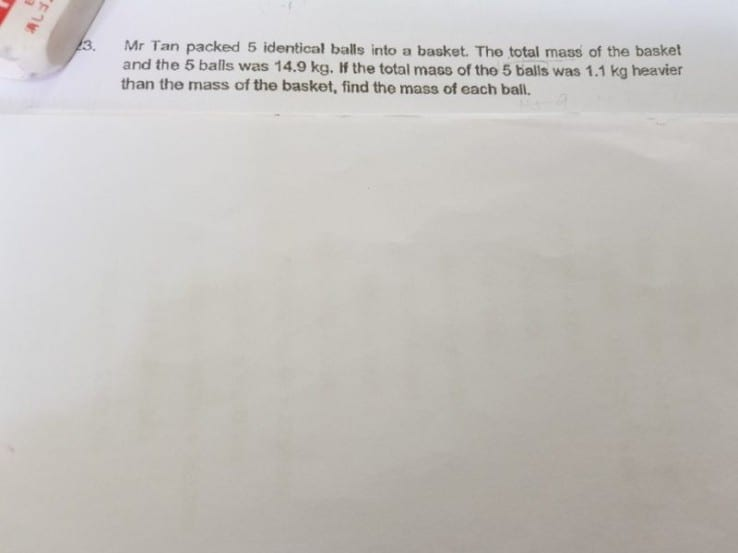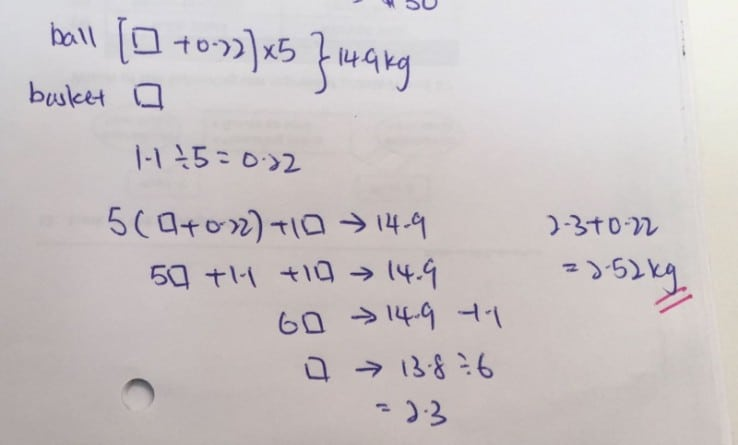QuestionHi,Since the total weight of the 5 balls is 1.1kg heavier than the weight of the basket:
14.9kg – 1.1kg = 13.8kg

The weight of the basket will be:
13.8kg/2 = 6.9kg

The total weight of the five balls will be:
6.9kg + 1.1kg = 8kg

The weight of one ball will be:
8kg/5 = 1.6kg.

0 Replies 1 Like1 Reply 0 Likes